#### · Potential (or stored energy)

Kinetic Energy…is energy in motion.
Any object in motion is using kinetic energy. For example, when you throw a baseball – when the baseball is “moving” that’s the kinetic energy. Moving water and wind are examples of kinetic energy. Electricity is kinetic energy because even though you can't see it happen, electricity involves electrons moving in a conductor.
Potential Energy… is stored energy (or latent).
Potential energy is stored energy that can then be converted to kinetic energy. A gallon of gasoline or a barrel of oil contains stored energy that can be released when we burn it. A lake in the mountains contains "stored" energy because, if it were released, it would do a lot of work on objects caught in its path. When we talk about oil, coal, wood, or gas, we are talking about "stored" energy.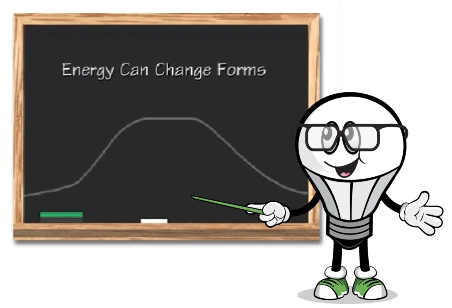Kinetic energy and potential energy can change forms.  For example, the car moving up the hill is kinetic energy.  When the car reaches the top of the hill and is about to go down the hill, that is the potential energy. When the car moves forward down the hill it is then again kinetic energy.

The 5 Types of Kinetic Energy: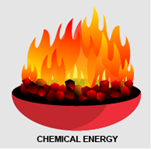Chemical Energy is the chemical reaction causing changes. Things like batteries, gasoline, and other fuel sources, and even food all store chemical energy. For example, when we burn coal the chemical energy is released and is converted to heat, also called thermal energy and light energy.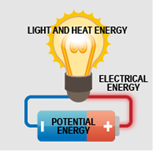Electrical Energy is when motion, light or heat is produced by an electrical current like from a battery which has stored electrical potential energy.  When the current is in motion it creates electrical energy.  Another example is the electric coils on a stovetop.  When the electric current moves through the coils it generates heat.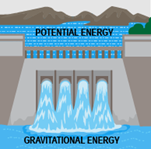Gravitational Energy is the energy an object has due to a change in its position. Water in a lake behind a dam has potential energy because it is higher than the stream on the other side of the dam. When gravity pushes the water through the gates at the bottom of the dam, energy is released – gravitational energy. Once the water gets down the hill, it has lost much of its gravitational energy.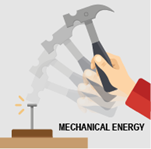Mechanical Energy is the sum of the potential and kinetic energy of motion and position in an object that is used to do the work. For example, energy from the wind turning a windmill, or the force of water turning a water wheel. When you swing a hammer to hit a nail, the action of swinging the hammer up then down is the kinetic energy, the hammer is the potential energy, so when combined to hit the nail, that is mechanical energy.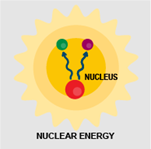Nuclear Energy is the energy in the nucleus of an atom. When these atoms are split by the process of nuclear fission, it creates a chain reaction and it releases energy in the form of heat.

The 5 Forms of Potential Energy: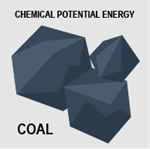Chemical Potential Energy is the energy stored within the bonds of atoms and molecules in a chemical substance. When the bonds are broken it creates a chemical reaction.  For example, fossil fuels like coal (the potential energy)  when burned, release heat energy.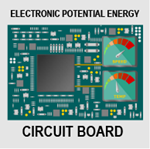Electrical Potential Energy is energy stored in an electric field. This energy is then set in motion by an electrostatic force, then that energy can become kinetic. Electronic circuit boards, for example, must be able to store energy in order to transfer it to a form of energy like light, heat or motion.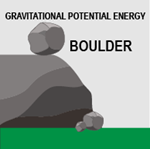Gravitational Potential Energy is energy that is stored in an object suspended above the height of zero, like a ground floor. The object or mass is in a gravitational field. When the object falls from its resting position, the energy is then converted to kinetic energy as it is falling. The higher the object has to fall, the greater the gravitational potential energy is.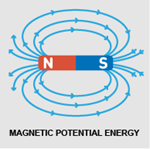Magnetic Potential Energy is the potential energy connected to the positions of magnetic objects. As you know, magnets have two poles, a North and a South pole. Opposite poles attract and like poles repel. So, the closer the opposite poles are, the more the potential energy will be stored and the magnets will attract to each other. Same with the like poles, the closer they are the more potential energy is stored to repel each other away.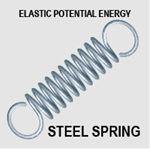Elastic Potential Energy is the compression or stretching of an elastic object. The potential energy amount depends on how much the object has changed in the amount of stretching or compressing. For example, when a spring is compressed a lot, it stores more potential energy than when it is only compressed a little.  When you release the spring that potential energy becomes kinetic energy.